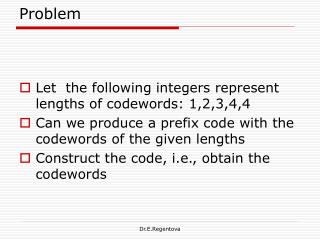DownloadDownload PresentationProblem

# Problem

Télécharger la présentation## Problem

- - - - - - - - - - - - - - - - - - - - - - - - - - - E N D - - - - - - - - - - - - - - - - - - - - - - - - - - -
##### Presentation Transcript

1. Problem • Let the following integers represent lengths of codewords: 1,2,3,4,4 • Can we produce a prefix code with the codewords of the given lengths • Construct the code, i.e., obtain the codewords Dr.E.Regentova

2. Solution • Check Kraft-McMillan’s inequality. If holds, then an instantaneous prefix code can be designed. • Design a binary tree with a number of levels given by the maximum length of codewords in the list. • Each time you designate a node in the binary tree for a codeword, truncate the tree, i.e., make the node a leaf node. • Traverse the tree from its root to the leaves assigning to the left move (branch) 0, and to the right move (1) or v.v. Dr.E.Regentova

3. Huffman Coding: Optimum Prefix codes The optimal code for a source S has an average length lav Dr.E.Regentova

4. Assumptions • Symbols that occur more frequently will have shorter codewords. • Lemma: In an optimum code, two symbols that occur least frequently will have the same length. • Two less probable symbols of the alphabet differ in the last bit. Dr.E.Regentova

5. Design • Consider the following alphabet P(a1)=P(a3)=0.2; P(a2)=0.4;P(a5)=0.1 • Sort them by descending order of probabilities. Dr.E.Regentova

6. Design • For two less probable symbols assign codewords as C(a4)=β1*0 (1) C(a5)=β1*1, where * is concatenation. • Combine two least probable symbols into one (denote a45)with a probability being equal to the sum of probabilities of these two symbols. This way, we design a new alphabet. • Resort the list. Dr.E.Regentova

7. Design Dr.E.Regentova

8. Design • This time, two less probable symbols are assigned c(a3) = β2*0 c(a45)= β2*1 • But, c(a45)= C(a4)+ C(a5)= β1*0+ β1*1= β1, hence β1 = β2*1 • We can rewrite c(a4)= β1*0= (β2*1) *0= β2*10 c(a5)=β1*1= (β2*1) *1= β2*11 • Combine again to obtain a new symbol a345 in the new alphabet. Resort the list. Dr.E.Regentova

9. Design Dr.E.Regentova

10. Design • Combine again two less probable symbols, i.e, a345 and a1, assigning c(a3451)= β3 • Then c(a345)= β3*0= β2 c(a1)=β3*1 • We can rewrite codewords as c(a3) =β2*0 = (β3*0)*0= β3*00 c(a4)= β2*10= β3*010 c(a5)=β2*11= (β3*0) *11= β3*011 c(a1)=β3*1 Dr.E.Regentova

11. Design A’’ Since there are only two letters is the alphabet, we assign β3=0, and c(a2)=1 Dr.E.Regentova

12. Design • Assign c(a3451)= β3 = 0 c(a2)=1 c(a1)= β3 *1=01 c(a3) = β3*00=000 c(a4)= β3*010=0010 c(a5)= β3*011=0011 Dr.E.Regentova

13. Properties of the code • Lav = 2.2b/letter • H= 2.278 b/letter • Redundancy :R=0.078 b/symbol • Variance ? Dr.E.Regentova

14. Code 2: • When resorting probabilities, put the combined letter always before any other letter of the same probability. • This way, the corresponding code will be of a lower variance. Dr.E.Regentova

15. Design 2: Minimum variance Instead of Obtain this Dr.E.Regentova

16. Building Huffman codes:2 Dr.E.Regentova

17. The algorithm Step 1: G+H = H 1 = 0.010; Step 2: E+F = F1 = 0.018 Step 3: F1 + H 1 = H 11 = 0.028;Step 4: H 11 + D = H 111 = 0.109 Step 5: B+C = C1 = 0.162; Step 6: H 111 + C1 = H 1Y =0.271 Dr.E.Regentova

18. Traversing Huffman Tree Dr.E.Regentova

19. Resulted Code Dr.E.Regentova

20. Properties of the resulted code l = 1.6 bits/symbol H= 1.22 b/symbol Redundancy: 0.62b/symbol Dr.E.Regentova

21. Code rate for the Huffman code For Huffman code, it is proved that if Pmax ≥ 0.5, the upper bound is H(S) +Pmax; If Pmax ≤, 0.5, the upper bound is H(S) +Pmax+0.086; Dr. E.Regentova